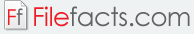### Math-o-mir 1.82

#### Danijel Gorupec (Freeware)

Math-o-mir is a program used for typing out mathematical expressions. The program was first released in 2009. It can be used as an alternative to using graph paper when dealing with mathematical expressions and equations. This program is also useful for teachers and students who want to do mathematical tasks on the computer. The application’s interface is similar to that of a word processor, except this one is used for equations. The application allows users to type in equations and add illustrations and drawings to support the equation as well.

Here are the other features of the Math-o-mir program:

• Support for plotting and analyzing functions and equations
• Mathematical equations can be edited using the mouse and keyboard
• Work created with the application can be exported as LaTeX code or as a raster image
• Comes with a wide variety of mathematical symbols and expressions
• Simple and intuitive user interface

Math-o-mir is a program that was developed for both novice and advanced computer users. It also comes with an autosave feature that ranges from never to high frequency. This makes sure that all changes made to the document are saved once in a while. The application also provides a wide variety of settings for all the user’s needs.